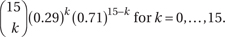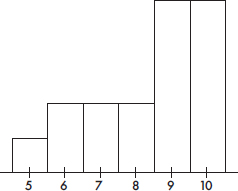# AP Statistics Multiple-Choice Practice Test 38

### Test Information10 questions23 minutes

1. To determine the mean cost of groceries in a certain city, an identical grocery basket of food is purchased at each store in a random sample of ten stores. If the average cost is \$47.52 with a standard deviation of \$1.59, find a 98% confidence interval estimate for the cost of these groceries in the city.

2. A set consists of four numbers. The largest value is 200, and the range is 50. Which of the following statements is true?

3. A telephone survey of 400 registered voters showed that 256 had not yet made up their minds 1 month before the election. How sure can we be that between 60% and 68% of the electorate were still undecided at that time?

4. Suppose we have a random variable X where the probability associated with the value k isWhat is the mean of X?

5. The financial aid office at a state university conducts a study to determine the total student costs per semester. All students are charged \$4500 for tuition. The mean cost for books is \$350 with a standard deviation of \$65. The mean outlay for room and board is \$2800 with a standard deviation of \$380. The mean personal expenditure is \$675 with a standard deviation of \$125. Assuming independence among categories, what is the standard deviation of the total student costs?

6. Suppose X and Y are random variables with E(X) = 312, var(X) = 6, E(X) = 307, and var(Y) = 8. What are the expected value and variance of the random variable X + Y?

7. In sample surveys, what is meant by bias?

8. The following histogram gives the shoe sizes of people in an elementary school building one morning.Which of the following is a true statement?

9. When comparing the standard normal (z) distribution to the t-distribution with df = 30, which of (A)-(D), if any, are false?

10. Given a probability of 0.65 that interest rates will jump this year, and a probability of 0.72 that if interest rates jump the stock market will decline, what is the probability that interest rates will jump and the stock market will decline?